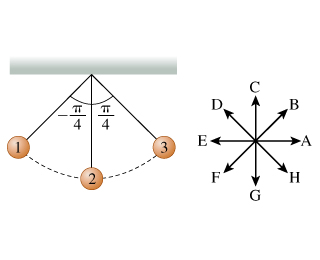# Problem: Which of the following is a true statement about the acceleration a→of the pendulum bob?A. a→ is equal to the acceleration due to gravity.B. a→ is equal to the instantaneous rate of change in velocity.C.a→ is perpendicular to the bob's trajectory.D. a→is tangent to the bob's trajectory.

###### FREE Expert Solution

The acceleration is maximum at positions 1 and 3 when the displacement is maximum. At position 2, the acceleration is zero because the pendulum is in the equilibrium position (there is no net force acting on it).

93% (171 ratings)###### Problem DetailsWhich of the following is a true statement about the acceleration $\stackrel{\mathbf{\to }}{\mathbf{a}}$of the pendulum bob?

A. $\stackrel{\mathbf{\to }}{\mathbf{a}}$ is equal to the acceleration due to gravity.

B. $\stackrel{\mathbf{\to }}{\mathbf{a}}$ is equal to the instantaneous rate of change in velocity.

C.$\stackrel{\mathbf{\to }}{\mathbf{a}}$ is perpendicular to the bob's trajectory.

D. $\stackrel{\mathbf{\to }}{\mathbf{a}}$is tangent to the bob's trajectory.